# THE RELATIONSHIP OF MATTER AND ENERGY AND THE APPLICATION TO THE FIRST CAUSE

by George Williams < [email protected] >

## INTRODUCTION TO MODEL

The following is a model for the formation of the universe and the relationship of matter and energy. It is my belief that the classic models are lacking and do not explain certain aspects of subatomic and photon behavior.

Scientist have proven that matter stores energy and that E=MC² is true for the loss of mass proportional to energy expended. The force of nuclear reaction and the amount of energy stored in a single atom cannot not be explained by the subatomic models. If you think about how this much energy is stored in the form of matter, the answer must be rotating structural form. Solid matter can store energy and remain stationary and stable with regard to axial position if it is rotating. i.e. flywheel.  Matter is literally an energy force field or structured energy. The idea of thinking in terms of structured energy is difficult since we tend to perceive energy or force as nothing but a cause and can only be measured by its effect on matter. Due to the difficulty imagining energy as a massless effect; I tend to imagine air, water or objects with mass such as a straw or balloon. Since every part of the this dimension is filled with energy, we exist in an ocean of energy.

Consider the Universe. Science would have us believe that space is void. But space is only void of concentrations of structured energy that we know as matter. From the standpoint of energy, or magnetic and gravitational force space is full. The planets, stars, black holes, and quasars all follow rules of motion. Forces act on forces and forces acting on stars and planets can hardly be considered void or nothingness. In fact everything affects everything to some extent.

Since energy is a difficult concept let us suppose that the universe is filled with water. For example, imagine a small eddy in water. The eddy currents have a hollow center and could not resist the flow of air through it but from any side it would have properties of mass. With regard to energy, only a single Axis of rotational structure would have length and width. So we can say that an energy eddy with a single axis (y) of rotation (acceleration) would only have two dimensional resistance to a lineal energy or force. (admittedly the eddy has height width and depth and are therefore three dimensional, but I’m talking in terms of structured energy.) Since pure energy cannot be measured except by and effect on matter or mass, let’s say it is a Y structure based on Cartesian coordinates.

Photon “And God said, Let there be light”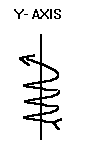Now consider a photon. If a photon were an energy eddy with a single axis of rotation, it would have properties of matter and energy. It would have a single axis of rotation; therefore having direction and frequency or wave form. It would be effected by magnetism and gravity. It could be created by electrons tumbling and interacting in a concentrated magnetic field. The photon would vary with the diameter of the rotation. The cause and effect of the fields that create it would determine its diameter or frequency.

If one imagines the rotating field or energy eddy on a plane the rotation would cause motion. It would have direction and frequency. Think of a tire rolling down a hill. It has both physical and wave properties. Is light a wave or matter? The answer is yes. It is both. Because a photon is the most basic component of structured energy. During creation of this time space dimension it would be the first thing created. This is shown in the Y Structure (Ys) diagram to the left.

I know they say that a photon has no mass, but they used to say that about electrons also. Photon mass is let us say a property that allows it to be affected by gravity and magnetism.

Electron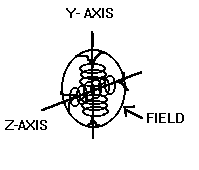What if this spinning group of Y’s or energy is spun on both the vertical Y horizontal X direction of rotation; you would have a dual axis rotation (XY structure) (acceleration). Dual Axis of rotation would have three dimensions length, width and height. If the object spins fast enough it would take on the properties of a solid spherical object.  A two dimensional accelerating object is a model for the electron. The smallest stable mass. A rotational couple is formed when two directions of rotation are joined. We have both mass and stable wave form.

The field or structure is fixed. It is the smallest fixed particle of mass. I know that somebody will say what about the quark. But the quark is not a fixed and independent structure. A quark is only a subcomponent of the structure’s field. Each polar region has a concentrated field that effect the electron microscope in a way that indicates structure.

Proton

How may directions can an object be rotated (or accelerated) at the same time ? Three XYZ Axis. Imagine this sphere rotating X and Y axis now spin it on the Z axis or third dimensional axis. A new property is formed, Mass/Gravitation Couple. Therefore rotating energy has Measurable Mass/Gravity. (while electrons have mass it is small compared to proton ) The third axis of rotation (or acceleration ) gives you mass or E=MC2.  Measurable Mass /gravity only equals the last property of acceleration and therefore relates to the formula for centripetal force and gravitation.

Gravity is the constant impelling force associated with mass. Mass is created by the outward pushing centripetal force and gravity is the impelling force. Gravity is directly proportional to mass. This presents the possibility that a change in the apparent mass will also cause a change in the gravitational effect.

To review, a particle with a single axis of rotation is a photon. It has field mass singular rotation, frequency and direction. It is created by the collision of opposing fields of rotation. Its frequency varies depending on the concentration of field in which it was created. An electron has two axis of rotation. It is a fixed and stable parcel, it has fixed mass and magnetic properties.

A proton has three axii of rotation. It has a larger mass and gravitational force based on the third direction of rotation. It stores energy as mass / gravity. The field’s structure by nature forms concentrations of energy that are quarks and anti-matter. Each axial field is made up of a quark and anti-matter property. While an electron also has the quark/anti-matter relationship, these properties are made more apparent by the third axis of rotation which expands area and amplifies the field effect.

## THEOREM

Based on the above description I would like to describe the events that formed matter. Please keep in mind that while I’m describing the events with regard to a single atom; the events were happening on a universal scale and that the time of the events is indeterminable since the event to time ratio was changing.

1) Let’s assume that just prior to creation of the universe that within the matterless and engeryless temporal / spatial dimension where our universe now exists the law of physics existed (no speed faster than light).

2) Next let’s assume that the force (first cause) which causes the big bang is from another adjacent temporal and spatial dimension. The bang begins from a small rupture in the time space continuum.

Imagine our universe as a balloon with nothing in it and the bang eruption as the air needle you insert to blow it up. The needle has no shaft so it is really like a infinitesimal doorway which grows larger as the energy flow increases and the new dimension expands. Let’s say the cause is from an implosion in an adjacent dimension sharing time but not space. (but only for the moment of the implosion) Event time must be shared but remember our dimensions aren’t sharing space. The air in the balloon doesn’t share space with the air outside of it. I would suggest that a super gravitation event in all four dimensions (length, width, height, and time) of the adjacent time space continuum caused the rupture.

3) As the energy is released from the dimensional doorway. Let’s assume that it increases directly proportional to time and the increasing tear in the adjacent dimension’s time space continuum. ET1<ET2<ET3<ET* WHERE ( E) =ENERGY (T) = TIME (1,2,3,) MARK THE CHRONOLOGICAL SEQUENCE OF THE EVENT AND A VALUE OF INCREASING LINEAL ENERGY ACCELERATION EMANATING IN ALL DIRECTIONS AT ONCE, ( *) BEING MAXIMUM ENERGY TO SEQUENCTIAL EXPANSION ACCELERATION (TO THE SPEED OF LIGHT).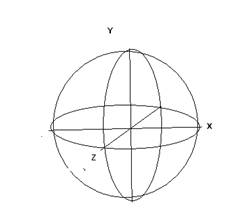The sequence RUPTURE <ET1<ET2<ET3<ET*=E-(TOTAL MATTER ) note the E- total matter (not mass) since all matter even to the smallest particle contains energy by the nature of its existence.  As the energy emanates and increases acceleration, the energy in front resists the change in acceleration of the energy behind it. It is the physical laws and limitations of the forming universe that form the resistance; therefore, without these laws of physics matter and/or mass could not be formed. As the ever increasing energy behind (ET2) pushes passed (ET1), it causes the less intense linear energy to form eddies (ET1). As ET1 begins to rotate it forms a field of resistance which has two physical dimensions width and depth. These energy eddies reach a maximum rotation/acceleration. Then the eddies take on the properties of matter on the Y axis of rotation but in the vortex of the eddy the properties on energy still exist. (photon). (The structure of a photon is not stable out side of a force field since the second dimensional axis of rotation is not established it dissipates and tends to have lineal direction of movement through other fields.) But for now our photon is still trapped and resisting acceleration. It is still being pushed from behind As the eddy resists the continued increase in energy and rotation/acceleration, the eddy begins to rotate on the perpendicular (X) axis to maximum rotation/acceleration. (It is now rotating on both the x and y axis and has properties to matter in a stable field in three dimensions without measurable properties of mass and two dimensional acceleration.)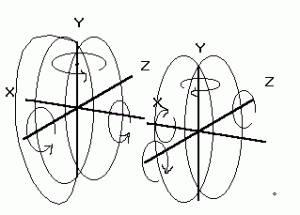The new particle is call E (electron). The E particle is further resistant to acceleration and the increasing magnitude of the energy (E*).Two particles of E with opposite polarity are force together and begin to resist the emanating energy and begins to rotate on the third axis or Z. The matter now takes on the properties of measurable mass. Proton/Neutron couple As the eddy resists the continued increase in energy

and rotation/acceleration, the eddy begins to rotate on the perpendicular (X) axis to maximum rotation/acceleration. (It is now rotating on both the x and y axis and has properties to matter in a stable field in three dimensions without measurable properties of mass and two dimensional acceleration.) The new particle is call E (electron).

The E particle is further resistant to acceleration and the increasing magnitude of the energy (E*).Two particles of E with opposite polarity are force together and begin to resist the emanating energy and begins to rotate on the third axis or Z. The matter now takes on the properties of measurable mass. Note: the tangent point has equal and opposite acceleration in all three planes of physical dimension. Plus and Minus symbols represent polar direction only as in a magnet.

As you can readily see matter is not destroyed when the atom is split ; rather measurable mass is converted to energy but your left with some really screwed up electrons.(If you could capture these wild electrons in a three dimensional magnetic field and stabilize the energy. What a power supply you would have) This is difficult to explain on a few pages.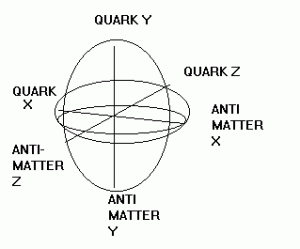Subatomic Particles and Antimatter

I think that while physicist’s try to discover subatomic particles they are seeing the concentrated areas around the positive poles of each axis and identifying these poles as quarks. The negative poles are being identified as antimatter. In some instances the process that physicists are using to view the sub atomic particles actually creates the particles (small photon type eddies) Its kind of like looking so close at the trees that you can’t find the forest. Since the area identified as quarks does not account for the entire volume and mass of matter; the remaining volume of mass is the field of stored energy. The field property that is matter and mass is missed.  Depending on the state of the surrounding field and whether the particle is in a state of stability the subatomic particles created will vary.

Gray Matter

If the so called big bang happened and matter formed according to my model the Gray Matter or missing matter is accounted for by the fields created by X,Y,Z rotation (three dimensional acceleration structure of energy in a state know as matter). You could for the sake of mathematics consider a structured pool of neutrinos.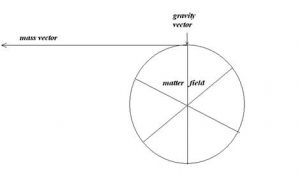Gravitation and Mass

Since opposing forces would be required to stabilize the third dimensional property which creates the effect of mass, the opposing force is gravity, the combined fields of the collective mass have a cumulative effect which results in gravitational force. Gravitation is a constant impelling force. The impelling force is offset by the centripetal force of the Z-axis of rotation or third property of acceleration. Gravity and Mass can be viewed as the vector sum of the matter field effect. Mass, and Gravity are properties of the force field that is matter.  Lets suppose that we have a little water in a hollow globe. If we rotate the globe on a single axis (call it X) the water flows to the equator of the globe. If we rotate the globe on the another axis Y at the same time, the water would cover the inside of the sphere with equal pressure at every point. Now if you rotate the globe on a third axis Z at the same time such that X, Y and Z share a single common center point, then this additional energy is displaced as mass and gravity.

Electro- Magnetism

Electrons have three dimensional properties of matter without measurable mass / gravity (virtually no gravity). They have two dimensional structured acceleration or rotation. Each electron rotate on the X/Y axis. When electrons flow in a path such as a coil the relative polarization of one of the two axii of acceleration are aligned the lineal path creates a relative cumulative polarization. The polarization magnifies the effect of the plus minus x axis of rotation. An electron is the perfect motor. Up till now we have used only the transient properties of its’ field to do work. We use electrons like pressurized fluid. We align it , push it, and manipulate it to transfer mechanical energy. We have not tapped it as an energy source. I think that if electrons could be held in a force field, we could extract power directly.

Nuclear Energy and Anti-Gravity

When protons are split and the radio active particles are sent out, I think that acceleration of the radio active particle could be reduced by placing the radio active particles in a three dimensional force field. I believe that useable energy can be derived while accelerating the period of radioactive decay. If a coil were constructed which magnetically/electrostatically restricted the movement of the radioactive particles the resulting magnetic field in a secondary coil would cause electrical flow. The three dimension field coil is basically three electromagnets position in a way respectively to create a three dimensional force field. It consists of X, Y, and Z coils inside a static shield. These coils simulate mass which has a direct gravitational effect. This gravitational effect could be increased or decreased. The problem is that you need to extract enough energy from the field to generate its own magnetism. This is not however energy from nothing since you are accelerating a decay of mass in the radioactive material. This in turn accelerates the decay of the radioactivity. Photons are created when electrons are tumble within a field The resulting eddy is a single polar unstable reaction.

Instant travel

If we could move into the time adjacent dimensional time space continuum then we could emerge back again into our own dimension we could travel vast distances of space in an instant. It is conceivable that consciousness is our link to the vast dimension outside of this continuum. The realm of Jung’s collective unconsciousness, The twilight zone.

Black Hole

The formation of our time space continuum is similar to the description associated with a black hole. In another dimension a black hole exists or existed energy and mass imploding until no mass exists in the time space dimension where the cause took place but energy / mass is displaced in the newly formed adjacent dimension. Black holes could be the opposite side of a big bang explosion. Black Holes could be creating other dimensional time space continuums right now.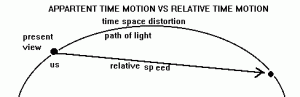TIME

Is time a constant or does it change as the events happen faster? Einstein’s Relativity simple stated that time is proportional to the speed of the event. But lets assume the duration of time that we perceive is constant measurement unrelated to the event. Then the age of the universe would be less than estimated. The speed of the expansion would have followed a parabolic curve increasing during the formation of mass , matter then slowing due to gravity. Our present expansion would be at the slowest rate ever. Though from our perspective on earth the view would appear to be increasing in speed since our view is through the bend in the time continuum we tend to see the distance like viewing down the opposite and adjacent sides of the right triangle, where true time speed is the hypotenuse. Thus while slowing it appears to accelerate. The Keep in mind that the appearance of star’s age would be equally distorted, since the time factor associated with the event would have changed proportional to time so that the appearance of stars would be older. Star’s ages are estimate using time / event constants. The only thing that science can study about energy is the effect of energy on matter in a time sequence. But we view time as a sequence of events or a sequence of changes on matter. The total scientific knowledge of man is base on measurement and properties of matter or events. We can now observe and measure only under the condition that now exists during the condition of forces that now exist. Through most of the formation of the universe the events were probably happening at a faster rate. Our whole idea about the age of the universe, stars and the earth is probably distorted. It is arbitrary to conclude since time is only relevant to the event. What happens in one second now was not what happened then in the time measurement we know as a second. If the speed of light is the maximum velocity then light can be the only true measure of time. I don’t think time is as much the variable as is matter and energy. What if time is constant and mass changes. We use objects of matter to measure time. Perhaps only our measure of time is distorted. If a second is only defined as the event perhaps seconds have changed. This explanation only serves to re-enforce the concept of Plato’s that the reflection on the wall of the cave is truly distorted.